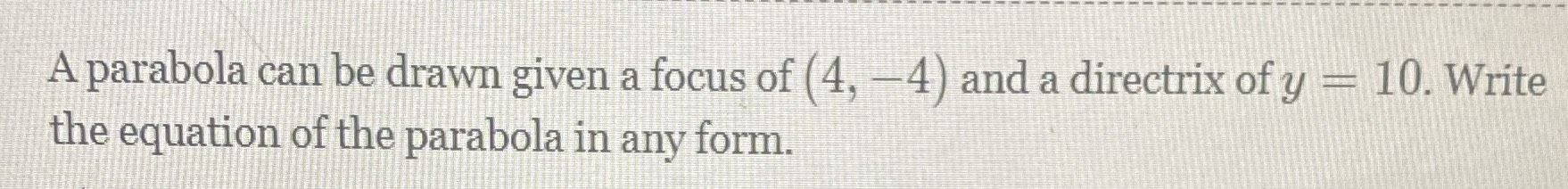### ¿Todavía tienes preguntas de matemáticas?

Pregunte a nuestros tutores expertos
Algebra
Pregunta

A parabola can be drawn given a focus of $$( 4 , - 4 )$$ and a directrix of $$y = 10$$ . Write the equation of the parabola in any form.$$y= - \frac{x^2}{28}+ \frac{2x}{7}+ \frac{17}{7}$$